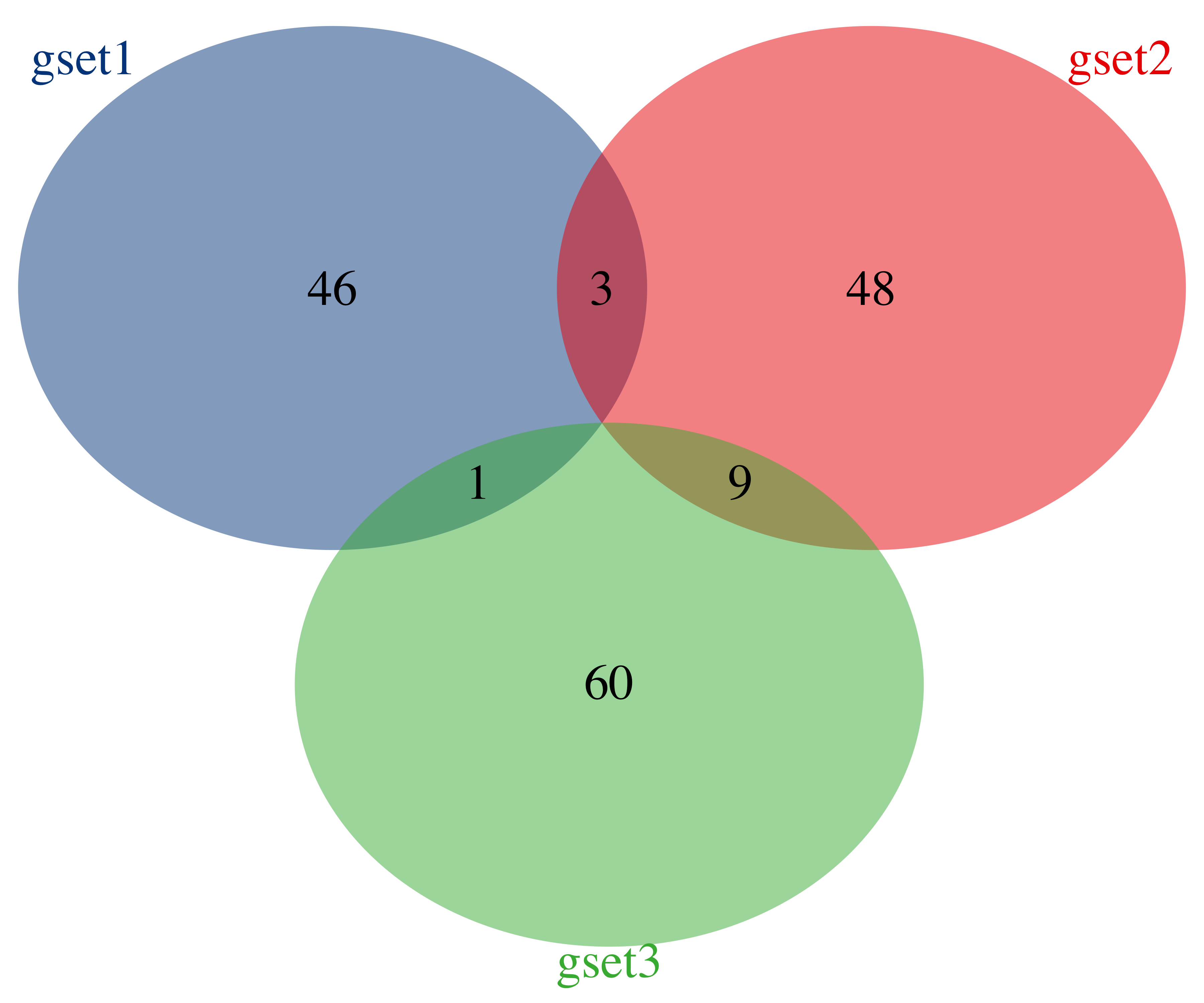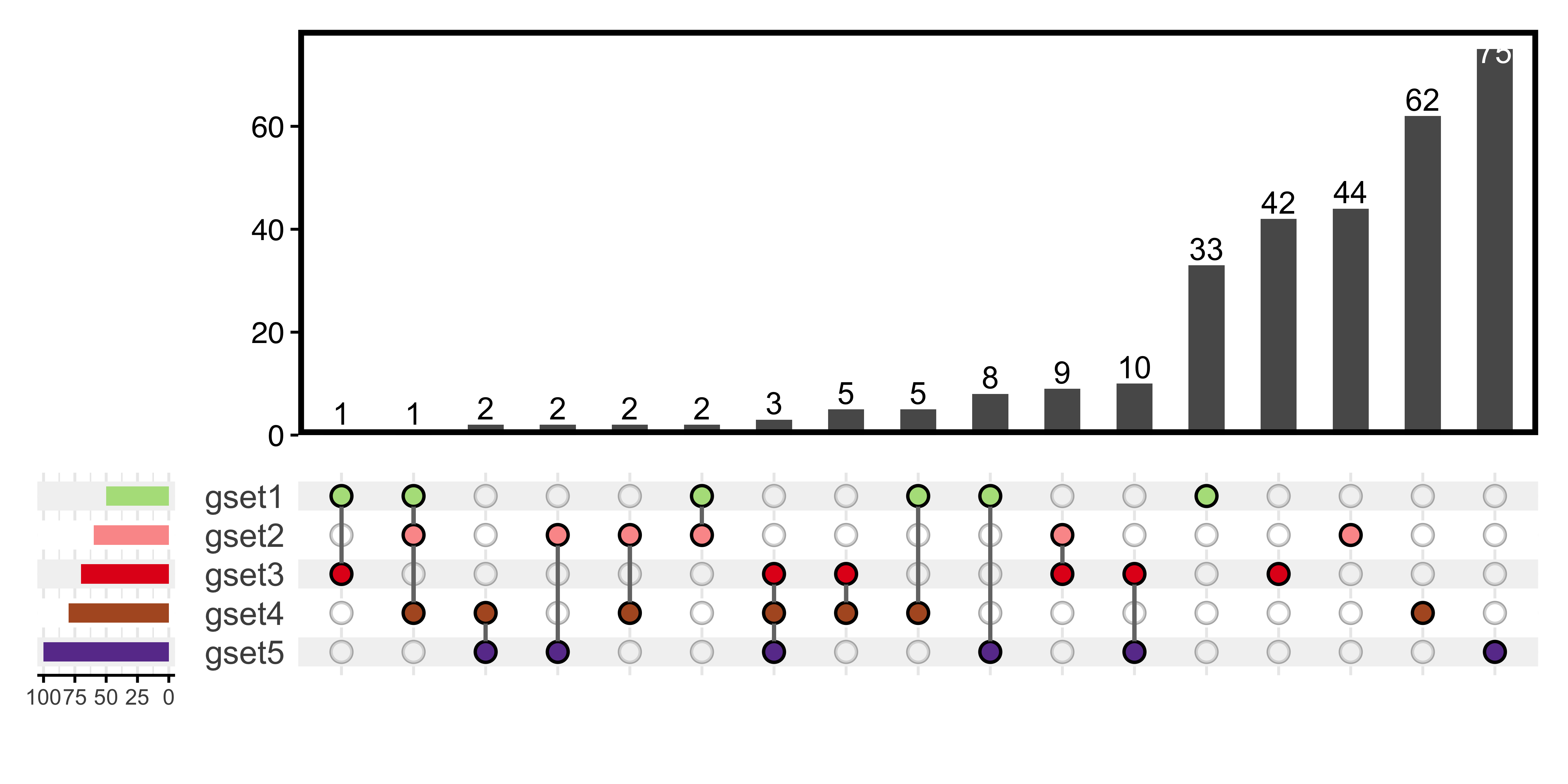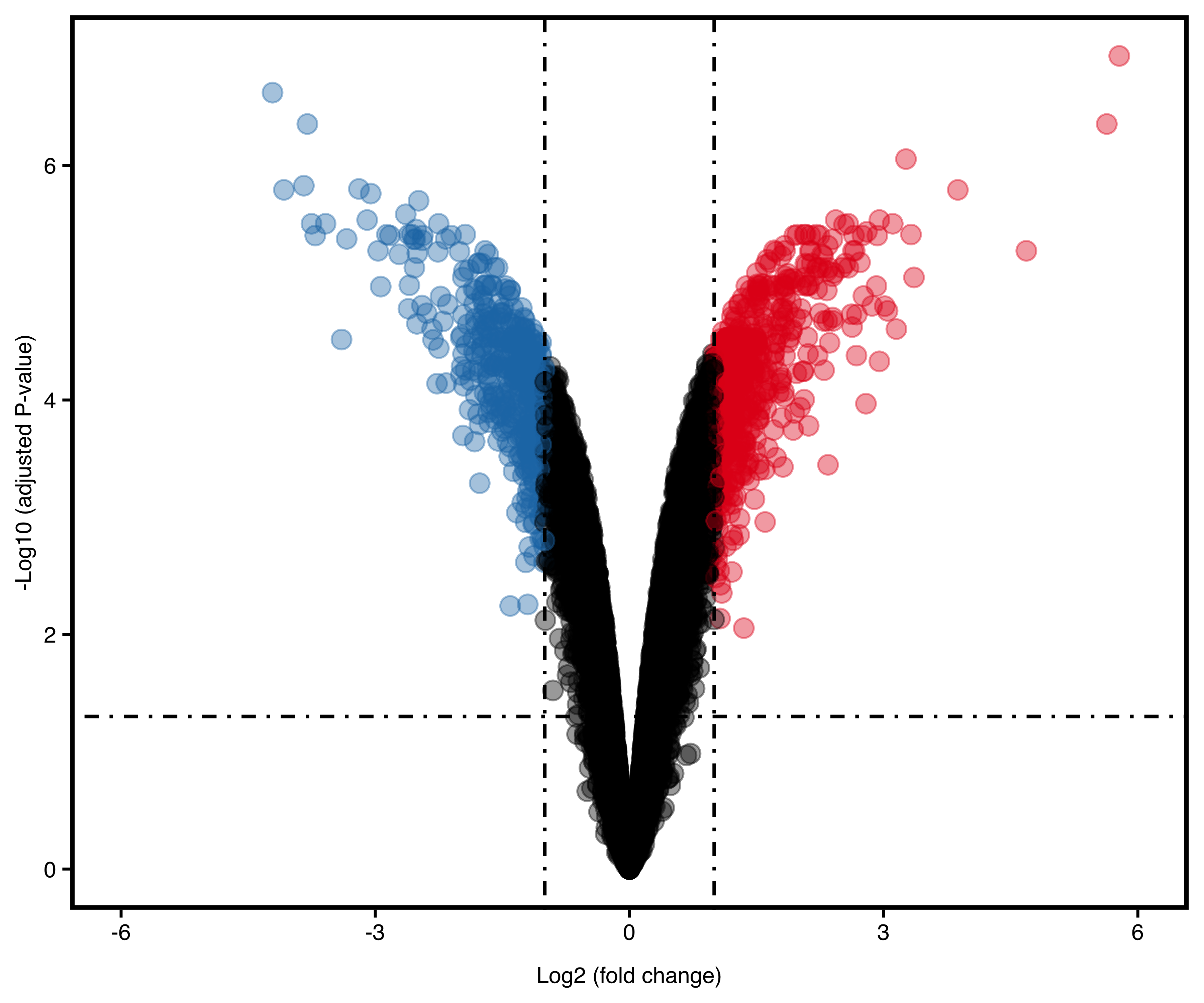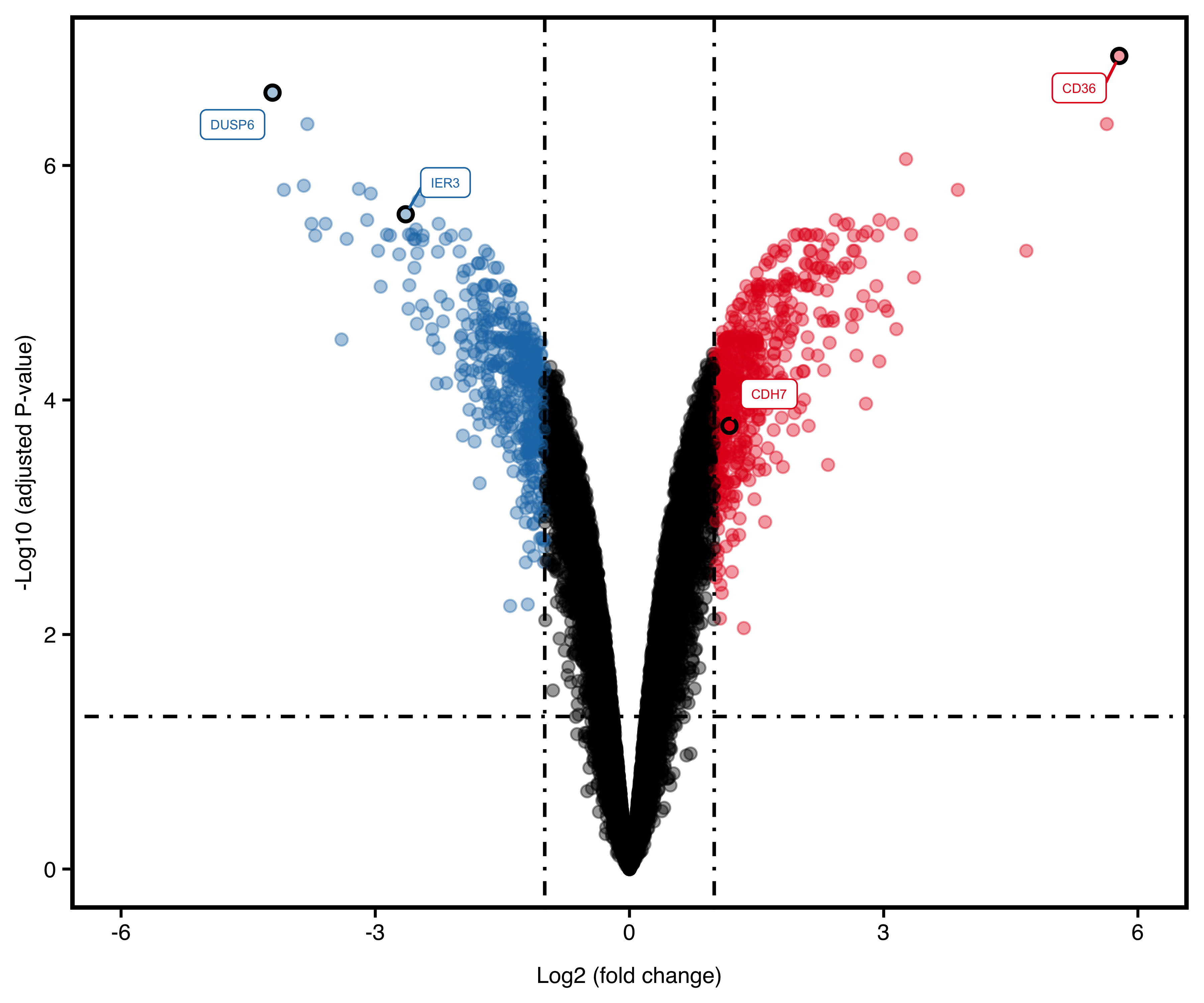# 13 Plot others

In this part, we will introduce `plotVenn` and `plotVolcano`.

## 13.1 Venn Plot

Venn plot needs a list of gene sets, for example:

``````set1 <- paste0(rep("gene", 50), sample(1:1000, 50))
set2 <- paste0(rep("gene", 60), sample(1:1000, 60))
set3 <- paste0(rep("gene", 70), sample(1:1000, 70))
set4 <- paste0(rep("gene", 80), sample(1:1000, 80))
set5 <- paste0(rep("gene", 100), sample(1:1000, 100))

# three groups
sm_gene_list <- list(gset1 = set1, gset2 = set2, gset3 = set3)

# five groups
la_gene_list <- list(
gset1 = set1, gset2 = set2, gset3 = set3,
gset4 = set4, gset5 = set5
)``````

`plotVenn` supports two types: Venn (default) and UpSet. Besides, it also supports modification of text size, alpha degree, colors and other parameters from `plot_theme`.

``````plotVenn(sm_gene_list,
use_venn = TRUE,
color = ggsci::pal_lancet()(3),
alpha_degree = 1,
main_text_size = 1.5,
border_thick = 0
)``````Figure 13.1: Venn plot

UpSet plot is better for over four groups of genes:

``````plotVenn(la_gene_list,
use_venn = FALSE,
main_text_size = 15,
legend_text_size = 8,
legend_position = 'left'
)``````Figure 13.2: UpSet plot

## 13.2 Volcano Plot

After finishing differential expression analysis, we could get differentially expressed genes (DEG). Volcano plot is widely used for these up/down regulated genes visualization.

The example data is micro array differential expression analysis:

``````data(deg, package = "genekitr")
``````##   probe_id      logFC   AveExpr         t      P.Value   adj.P.Val          B
## 1  8144866  0.5672300  5.761898  6.591099 2.496346e-04 0.002269049  0.3372158
## 2  8066431  0.6526467  9.809843  7.768724 8.554137e-05 0.001045237  1.5161421
## 3  8022674  0.2768667 11.926333  3.958127 4.974269e-03 0.021521339 -2.9321234
## 4  7925531 -0.1321333 11.217700 -1.914468 9.517321e-02 0.198247263 -5.9932047
## 5  8081358  0.3972133  8.994403  4.457386 2.618820e-03 0.013189962 -2.2375610
##   change  entrezid     symbol
## 1 stable        10       NAT2
## 3 stable      1000       CDH2
## 4 stable     10000       AKT3
## 5 stable 100009676 ZBTB11-AS1``````

`plotVolcano` mainly uses a DEG result which should include gene id, fold change and statistic value.

It also has other parameters:

• `stat_metric`: choose from “pvalue” and “p.adjust”
• `stat_cutoff`: cutoff for significant statistic value, default is 0.05
• `logFC_cutoff`: cutoff for log2 (fold change), default is 1 which is actually two fold changes
• `up_color`: color for up-regulated genes
• `down_color`: color for down-regulated genes
• `show_gene`: show some gene names, default is none. Suggest renaming the column with these genes as “gene”.
• `dot_size`: volcano dot size
``plotVolcano(deg, "p.adjust", remove_legend = T, dot_size = 3)``Figure 13.3: Volcano plot

If we want to show some genes:

``````plotVolcano(deg,
remove_legend = T,
show_gene = c("CD36", "DUSP6", "IER3", "CDH7")
)``````Figure 13.4: Volcano plot with genes.

Trick for increasing text size:

User could hide all x/y-axis labels and raise `main_text_size` to enlarge gene text, then export the figure into PPT to add labels.

``````plotVolcano(deg,